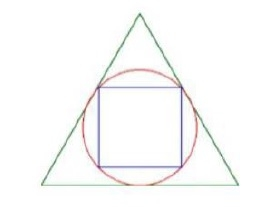# Area of a square inscribed in a circle which is inscribed in an equilateral triangle in C Program?

The program to find the area of a square inscribed in a circle which itself is inscribed in an equilateral triangle. The radius of circle inscribed inside an equilateral is a/(2√3).

The diameter of circle is the diagonal of square, d = 2 * r = a/ √3

Area formula for area of square given its diagonals is ½ d2

A = 0.5 * d2 A = (1/2) * (a2) / (3) = (a2/6)## Example

#include <iostream>
using namespace std;
int main() {
float area,a = 10;
area = (a*a) / 6;
cout <<"area = "<<area<< endl;
return 0;
}

## Output

area = 16.6667